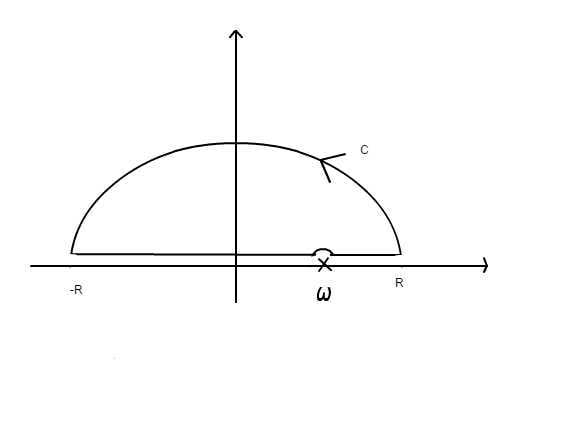# Locate the poles of the response function for an LRC circuit

## Homework Statement

Locate the poles of the response function $\alpha(\omega)$ in the complex plane for an LRC circuit.

## Homework Equations

$$\alpha(\omega)=\frac{-i\omega}{L}\frac{1}{\omega_0^2-\omega^2-i\omega\gamma}$$
$$\omega_0^2=\frac{1}{CL}$$
$$\gamma=\frac{R}{L}$$

## The Attempt at a Solution

So we've been going over how to locate poles and residues with normal functions and this is the first time doing something that is applicable to physics. Normally I'm used to having a function of x and changing this into a function of z and finding the poles that way. I'm not really sure how to start this question whether I should be changing ω to z or whether this is already a complex function. I've tried using the quadratic formula on the denominator in which I get

$$\frac{-\gamma \pm \sqrt{\gamma^2-4\omega_0^2}}{-2i}$$

but I do not think this is correct.

Any help would be nice.

Thanks

andrewkirk
Homework Helper
Gold Member
It looks Ok to me. Why don't you think your answer is correct?

##\omega## in the physical world will be a real number. But the formula you've given for ##\alpha(\omega)## doesn't require ##\omega## to be real.

As to what, if any, physical significance the two poles of the ##\alpha## function have - you'd need to ask an electrical engineer.

The reason I think it is wrong is because in the next part of the question it says:

ii) Show that for the contour C illustrated below, the integral of $\alpha(\omega)$/$\omega$ vanishes along the semicircular part of the contour as R goes to infinite.

I've attached what the contour looks like but in case it didn't work, it's a semicircle in the upper half of the plane and it shows a pole (marked as $\omega$) along the real positive axis. And the poles I found, if i did them correctly, should be on the imaginary axis.andrewkirk
Homework Helper
Gold Member
Are the poles relevant to that next part of the question?

I would have thought the asymptotic shrinking of the integral would be driven by the growth of R, rather than anything to do with the poles. Indeed, if the contour ran into a pole, that would blow up the integral, rather than shrink it.

What is a parametrisation of the points on the contour?

rude man
Homework Helper
Gold Member
I would think at this stage you'd have had the Laplace transform.
So for part (a), substitute s = jω (sorry, we EE's use j instead of i), then manipulate α(s) to look like (something)/(s + a). Then your pole is at s = -a.
As for part (b) the contour usually has σ for the x axis and jω for the y axis where s = σ + jω. I'm not quite sure what your plot is supposed to represent.